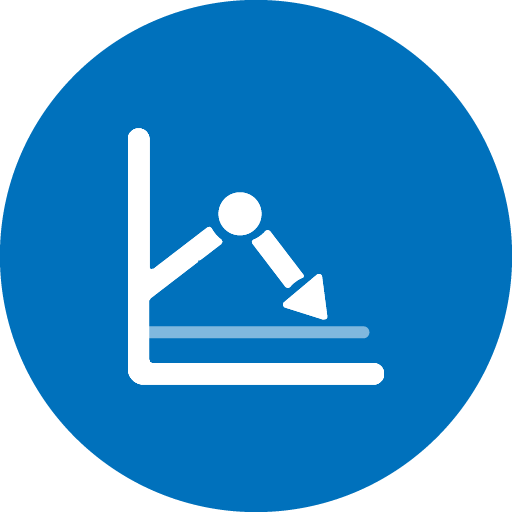#timeToTarget v1.3.7

#### timeToTarget Sensor

prediction of boundary crossing

##### Properties
• `tsaConfig` name of the Time Series Analytics configuration
• `target` threshold value that is checked
• `window` size of prediction window in seconds (default 3600). Alternatively you can use an iso8601 duration expression such as 'PT2H'
• `trend` 'up' if checking that predictions will be higher than target, 'down' if lower than target.

The to work on should be specified on the node. Use ‘\$’ if this resource should be the task resource.

When not set, direction depends on the last observation ('up' if target > last_observation)

• `confidenceLevel` confidence level of the prediction: indicates the minimal proportion of the prediction distribution that must cross the target.

`confidenceLevel=0.5` (default) can be used with all algorithms, and checks whether the (most likely) prediction crosses the target.

Other values for `confidenceLevel` check that target is crossed with that confidence (according to the confidence interval produced by the model!).

Currently only Arima supports confidence intervals for predictions.

By default (e.g. confidenceLevel=0.5)

• Crossed means that, according to the model, there is above 50% chance that the target might be crossed;
• Not Crossed hence means that this chance is below 50% (or likewise an above 50% chance that target is NOT crossed).

When low (e.g. confidenceLevel=0.1),

• Crossed means that the target might be attained (at least 1 in 10);
• Not Crossed means that there is above 90% chance that the target will NOT be attained

When high (e.g. confidenceLevel=0.9),

• Crossed means that the target most probably will be attained (more than 9 out of 10, according to the model);
• Not Crossed that there is above 10% chance that the target will NOT be attained

• `params` overrides for the analytics option configuration (see below)
##### States
• Crossed if it is predicted that values above (up) or below (down) the target will be attained ( with above `confidenceLevel` probability )
• Not Crossed otherwise
##### Raw data returned
• `target` the target value
• `crossed_at` observation at which the target is crossed first
• `confidence_level` confidence level used to decide whether the target might be attained
• `prediction_window`the size of the prediction window in which target crossings are checked
• `trend` up or down, depending whether predictions above or below the target were checked
• `extremal` maximal lower ('trend=up') or minimal upper ('trend=down') boundary of the (one-sided) confidence intervals of the predictions.

For c=0.5, this is the maximal ('trend=up') or minimal ('trend=down') prediction.

• `last_observation` last observation before the predictions
• `first_prediction` first prediction
• `last_prediction` last prediction

you must have TS Analytics package to run this sensor.

Before using this sensor, configure the analytics options for this metric using the Analytics Model Designer. Alternatively you should give a complete option configuration in the `params` section.

# Required properties:

• `tsaConfig`
• `target`
• `window`
• `trend`
• `confidenceLevel`
• `params`

# States:

• `Crossed`
• `Not Crossed`

# Raw data:

• `target (double)`
• `crossed_at (object)`
• `prediction_window (string)`
• `trend (string)`
• `extremal (double)`
• `last_observation (object)`
• `first_prediction (object)`
• `last_prediction (object)`
• `confidence_level (double)`